# COP 4710 Database Systems Spring 2006 Chapter 19

• Slides: 44COP 4710: Database Systems Spring 2006 Chapter 19 – Normalization – Part 3 Instructor : Mark Llewellyn [email protected] ucf. edu CSB 242, 823 -2790 http: //www. cs. ucf. edu/courses/cop 4710/spr 2006 School of Electrical Engineering and Computer Science University of Central Florida COP 4710: Database Systems (Normalization) Page 1 Mark Llewellyn ©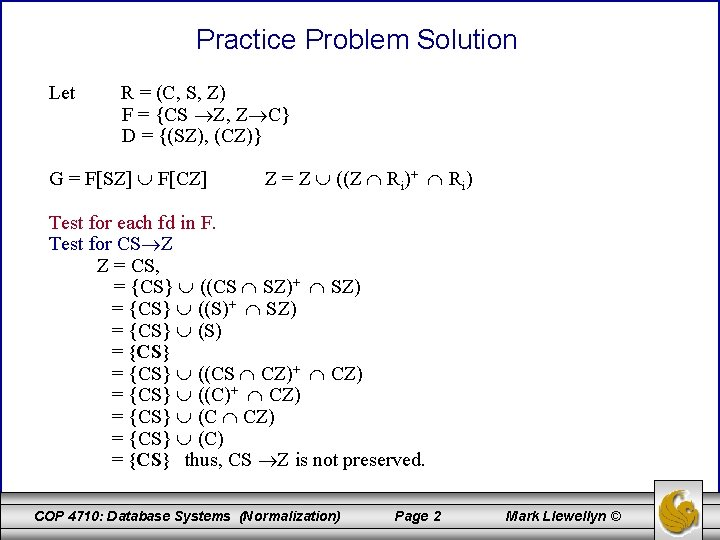Practice Problem Solution Let R = (C, S, Z) F = {CS Z, Z C} D = {(SZ), (CZ)} G = F[SZ] F[CZ] Z = Z ((Z Ri)+ Ri) Test for each fd in F. Test for CS Z Z = CS, = {CS} ((CS SZ)+ SZ) = {CS} ((S)+ SZ) = {CS} (S) = {CS} ((CS CZ)+ CZ) = {CS} ((C)+ CZ) = {CS} (C) = {CS} thus, CS Z is not preserved. COP 4710: Database Systems (Normalization) Page 2 Mark Llewellyn ©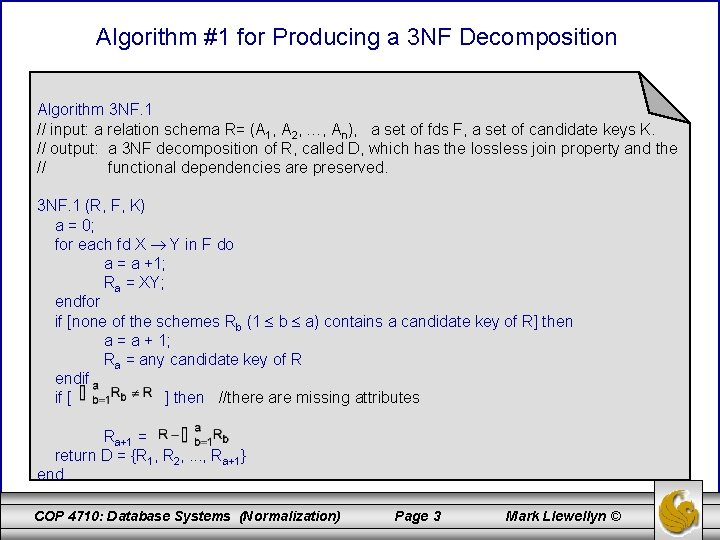Algorithm #1 for Producing a 3 NF Decomposition Algorithm 3 NF. 1 // input: a relation schema R= (A 1, A 2, …, An), a set of fds F, a set of candidate keys K. // output: a 3 NF decomposition of R, called D, which has the lossless join property and the // functional dependencies are preserved. 3 NF. 1 (R, F, K) a = 0; for each fd X Y in F do a = a +1; Ra = XY; endfor if [none of the schemes Rb (1 b a) contains a candidate key of R] then a = a + 1; Ra = any candidate key of R endif if [ ] then //there are missing attributes Ra+1 = return D = {R 1, R 2, . . . , Ra+1} end. COP 4710: Database Systems (Normalization) Page 3 Mark Llewellyn ©Example – Using Algorithm 3 NF. 1 Let R = (A, B, C, D, E) K = {AB, AC} F = {AB CDE, AC BDE, B C, C B, C D, B E} Step 1: D = {(ABCDE), (ACBDE), (BC), (CB), (CD), (BE)} Reduce to: D = {(ABCDE), (BC), (CD), (BE)} Step 2: Does D contain a candidate key for R? Yes, in (ABCDE) Step 3: Are all the attributes of R contained in D? Yes. Return D as: {(ABCDE), (BC), (CD), (BE)} COP 4710: Database Systems (Normalization) Page 4 Mark Llewellyn ©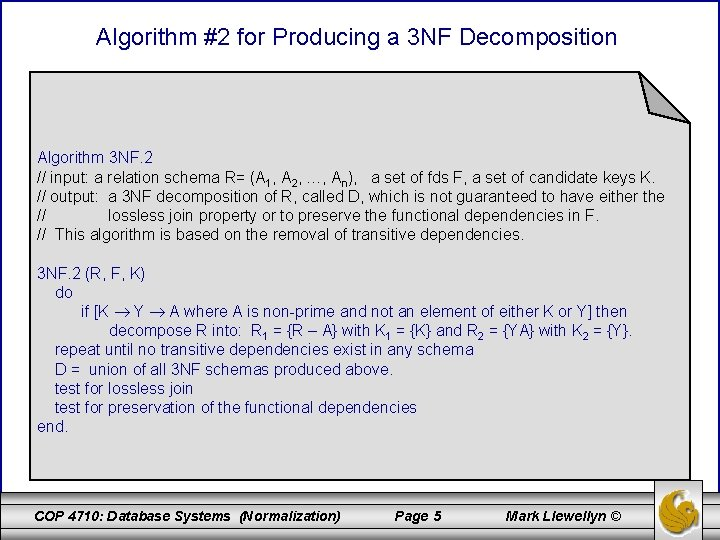Algorithm #2 for Producing a 3 NF Decomposition Algorithm 3 NF. 2 // input: a relation schema R= (A 1, A 2, …, An), a set of fds F, a set of candidate keys K. // output: a 3 NF decomposition of R, called D, which is not guaranteed to have either the // lossless join property or to preserve the functional dependencies in F. // This algorithm is based on the removal of transitive dependencies. 3 NF. 2 (R, F, K) do if [K Y A where A is non-prime and not an element of either K or Y] then decompose R into: R 1 = {R – A} with K 1 = {K} and R 2 = {YA} with K 2 = {Y}. repeat until no transitive dependencies exist in any schema D = union of all 3 NF schemas produced above. test for lossless join test for preservation of the functional dependencies end. COP 4710: Database Systems (Normalization) Page 5 Mark Llewellyn ©Example – Using Algorithm 3 NF. 2 Let R = (A, B, C, D, E) K = {AB, AC} F = {AB CDE, AC BDE, B C, C B, C D, B E} Step 1: R not in 3 NF since AB C D Decompose to: R 1 = (A, B, C, E) with K 1 = K = {AB, AC} R 2 = (C, D) with K 2 = {C} Step 2: R 2 in 3 NF. R 1 not in 3 NF since AB B E Decompose R 1 to: R 11 = (A, B, C) with K 11= K 1 = K = {AB, AC} R 12 = (B, E) with K 12 = {B} Step 3: R 2, R 11, and R 12 are all in 3 NF Step 4: Test for the lossless join property (see next page). COP 4710: Database Systems (Normalization) Page 6 Mark Llewellyn ©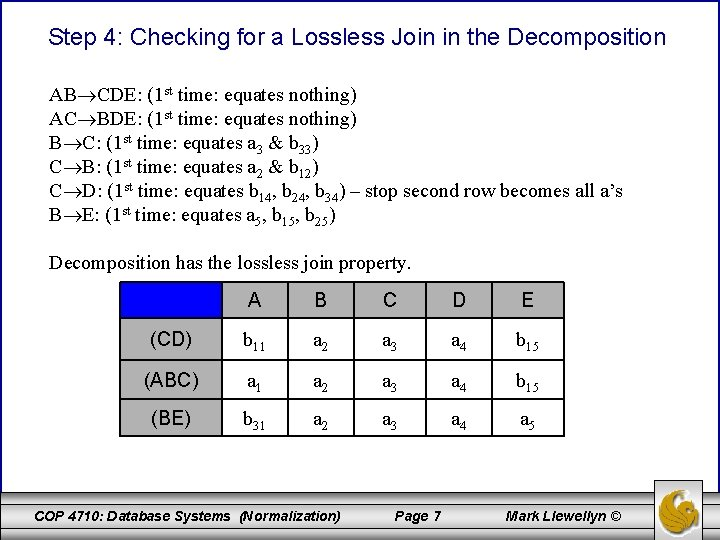Step 4: Checking for a Lossless Join in the Decomposition AB CDE: (1 st time: equates nothing) AC BDE: (1 st time: equates nothing) B C: (1 st time: equates a 3 & b 33) C B: (1 st time: equates a 2 & b 12) C D: (1 st time: equates b 14, b 24, b 34) – stop second row becomes all a’s B E: (1 st time: equates a 5, b 15, b 25) Decomposition has the lossless join property. A B C D E (CD) b 11 a 2 a 3 a 4 b 15 (ABC) a 1 a 2 a 3 a 4 b 15 (BE) b 31 a 2 a 3 a 4 a 5 COP 4710: Database Systems (Normalization) Page 7 Mark Llewellyn ©Step 5: Testing the Preservation of the Functional Dependencies Let R = (A, B, C, D, E) F = {AB CDE, AC BDE, B C, C B, C D, B E}} D = {(CD), (ABC), (BE)} G = F[CD] F[ABC] F[BE] Test for AB CDE Z = AB, = {AB} ((AB CD)+ CD) = {AB} (( )+ CD) = {AB} ((AB ABC)+ ABC) = {AB} ((AB)+ ABC) = {AB} (ABCDE ABC) = {AB} (ABC) = {ABC} ((ABC BE)+ BE) = {ABC} ((B)+ BE) = {ABC} (BCDE BE) = {ABC} (BE) = {ABCE} COP 4710: Database Systems (Normalization) Z = Z ((Z Ri)+ Ri) Page 8 Mark Llewellyn ©Step 5: Testing the Preservation of the Functional Dependencies (cont. ) Test for AB CDE continues Z = {ABCE} ((ABCE CD)+ CD) = {ABCE} ((C)+ CD) = {ABCE} (CBDE CD) = {ABCE} (CD) = {ABCDE} thus, AB CDE is preserved Test for AC BDE Z = AC = {AC} ((AC CD)+ CD) = {AC} ((C)+ CD) = {AC} (CBDE CD) = {AC} (CD) = {ACD} ((ACD ABC)+ ABC) = {ACD} ((AC)+ ABC) = {ACD} (ACBDE ABC) = {ACD} (ABC) = {ABCD} COP 4710: Database Systems (Normalization) Page 9 Mark Llewellyn ©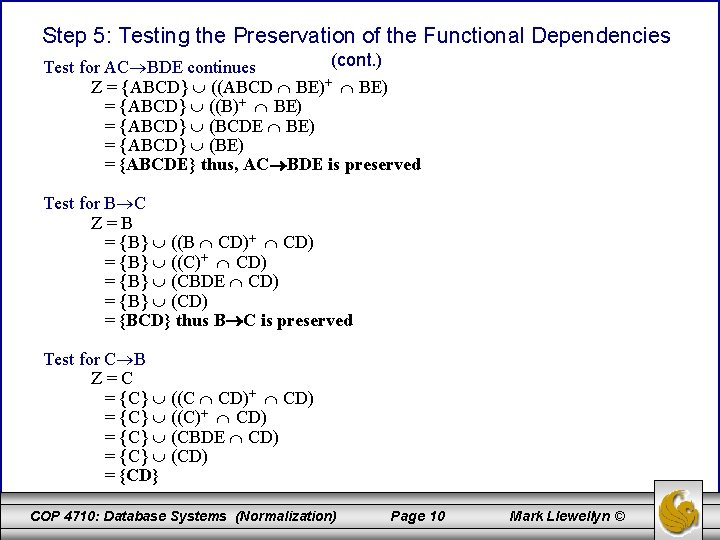Step 5: Testing the Preservation of the Functional Dependencies (cont. ) Test for AC BDE continues Z = {ABCD} ((ABCD BE)+ BE) = {ABCD} ((B)+ BE) = {ABCD} (BCDE BE) = {ABCD} (BE) = {ABCDE} thus, AC BDE is preserved Test for B C Z=B = {B} ((B CD)+ CD) = {B} ((C)+ CD) = {B} (CBDE CD) = {B} (CD) = {BCD} thus B C is preserved Test for C B Z=C = {C} ((C CD)+ CD) = {C} ((C)+ CD) = {C} (CBDE CD) = {C} (CD) = {CD} COP 4710: Database Systems (Normalization) Page 10 Mark Llewellyn ©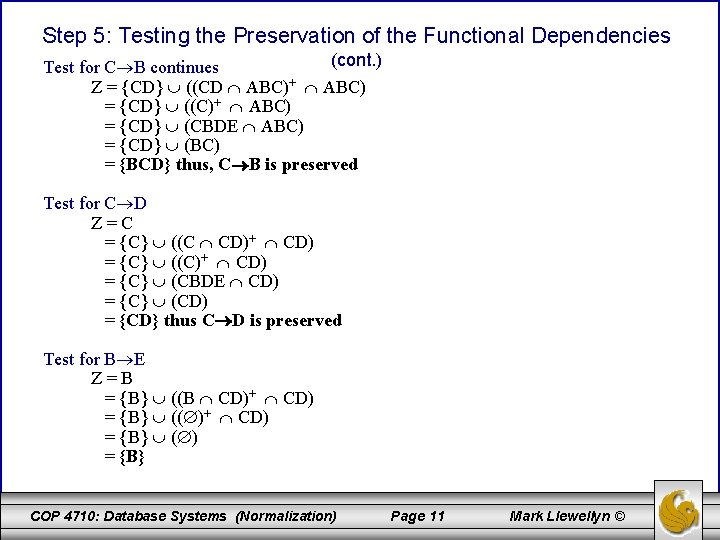Step 5: Testing the Preservation of the Functional Dependencies (cont. ) Test for C B continues Z = {CD} ((CD ABC)+ ABC) = {CD} ((C)+ ABC) = {CD} (CBDE ABC) = {CD} (BC) = {BCD} thus, C B is preserved Test for C D Z=C = {C} ((C CD)+ CD) = {C} ((C)+ CD) = {C} (CBDE CD) = {C} (CD) = {CD} thus C D is preserved Test for B E Z=B = {B} ((B CD)+ CD) = {B} (( )+ CD) = {B} ( ) = {B} COP 4710: Database Systems (Normalization) Page 11 Mark Llewellyn ©Step 5: Testing the Preservation of the Functional Dependencies (cont. ) Test for B E continues Z = {B} ((B ABC)+ ABC) = {B} ((B)+ ABC) = {B} (BCDE ABC) = {BC} (BC) = {BC} Z = {BC} ((BC ABC)+ ABC) = {BC} ((C)+ ABC) = {BC} (CBDE ABC) = {BC} (BC) = {BC} Z = {BC} ((BC BE)+ BE) = {BC} ((B)+ BE) = {BC} (BCDE BE) = {BC} (BE) = {BCE} thus, B E is preserved. COP 4710: Database Systems (Normalization) Page 12 Mark Llewellyn ©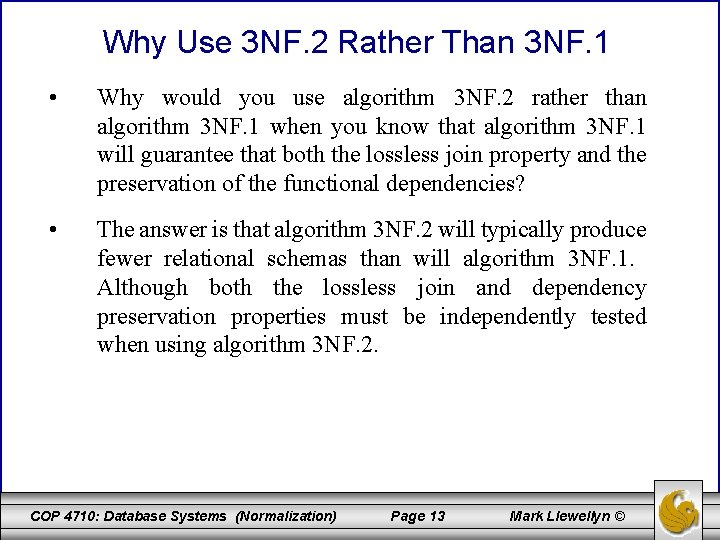Why Use 3 NF. 2 Rather Than 3 NF. 1 • Why would you use algorithm 3 NF. 2 rather than algorithm 3 NF. 1 when you know that algorithm 3 NF. 1 will guarantee that both the lossless join property and the preservation of the functional dependencies? • The answer is that algorithm 3 NF. 2 will typically produce fewer relational schemas than will algorithm 3 NF. 1. Although both the lossless join and dependency preservation properties must be independently tested when using algorithm 3 NF. 2. COP 4710: Database Systems (Normalization) Page 13 Mark Llewellyn ©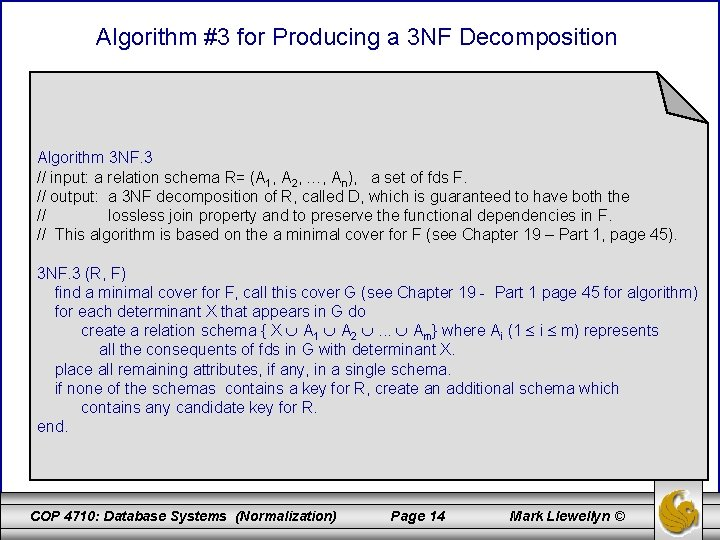Algorithm #3 for Producing a 3 NF Decomposition Algorithm 3 NF. 3 // input: a relation schema R= (A 1, A 2, …, An), a set of fds F. // output: a 3 NF decomposition of R, called D, which is guaranteed to have both the // lossless join property and to preserve the functional dependencies in F. // This algorithm is based on the a minimal cover for F (see Chapter 19 – Part 1, page 45). 3 NF. 3 (R, F) find a minimal cover for F, call this cover G (see Chapter 19 - Part 1 page 45 for algorithm) for each determinant X that appears in G do create a relation schema { X A 1 A 2 . . . Am} where Ai (1 i m) represents all the consequents of fds in G with determinant X. place all remaining attributes, if any, in a single schema. if none of the schemas contains a key for R, create an additional schema which contains any candidate key for R. end. COP 4710: Database Systems (Normalization) Page 14 Mark Llewellyn ©Algorithm 3 NF. 3 • • Algorithm 3 NF. 3 is very similar to algorithm 3 NF. 1, differing only in how the schemas of the decomposition scheme are created. – In algorithm 3 NF. 1, the schemas are created directly from F. – In algorithm 3 NF. 3, the schemas are created from a minimal cover for F. In general, algorithm 3 NF. 3 should generate fewer relation schemas than algorithm 3 NF. 1. COP 4710: Database Systems (Normalization) Page 15 Mark Llewellyn ©Another Technique for Testing the Preservation of Dependencies • The algorithm given on page 31 of Chapter 19 – Part 2 notes for testing the preservation of a set of functional dependencies on a decomposition scheme is fairly efficient for computation, but somewhat tedious to do by hand. • On the next page is an example solving the same problem that we did in the example on page 33 of Chapter 19 – Part 2, utilizing a different technique which is based on the concept of covers. • Given D, R, and F, if D = {R 1, R 2, . . . , Rn) then G = F[R 1] F[R 2] F[R 3] . . . F[Rn] and if every functional dependency in F is implied by G, then G covers F. • The technique is to generate that portion of G+ that allows us to know if G covers F. COP 4710: Database Systems (Normalization) Page 16 Mark Llewellyn ©A Hugmongously Big Example Using Different Technique Let R = (A, B, C, D) F = {A B, B C, C D, D A} D = {(AB), (BC), (CD)} G = F[AB] F[BC] F[CD] Projection onto schema (AB) F[AB] = A+ B+ (AB)+ = {ABCD} apply projection: = {AB} = {AB}, A B is covered Projection onto schema (BC) F[BC] = B+ C+ (BC)+ = {BCDA} {CDAB} {BCDA} apply projection: = {BC} = {BC}, C C is covered COP 4710: Database Systems (Normalization) Page 17 Mark Llewellyn ©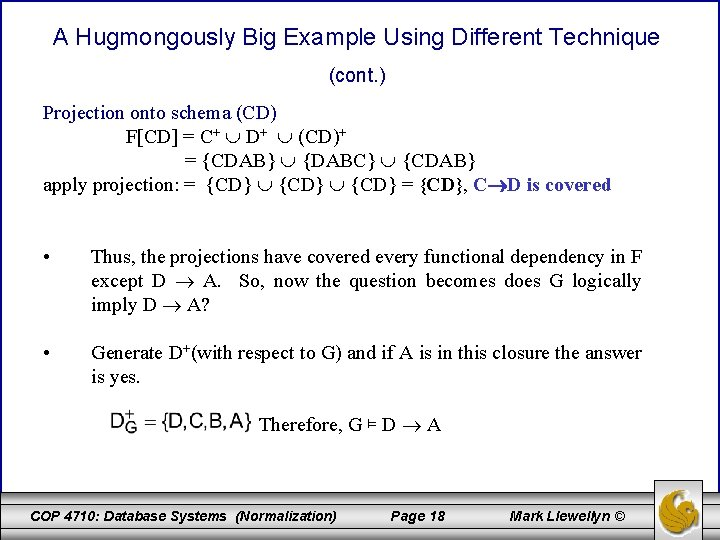A Hugmongously Big Example Using Different Technique (cont. ) Projection onto schema (CD) F[CD] = C+ D+ (CD)+ = {CDAB} {DABC} {CDAB} apply projection: = {CD} = {CD}, C D is covered • Thus, the projections have covered every functional dependency in F except D A. So, now the question becomes does G logically imply D A? • Generate D+(with respect to G) and if A is in this closure the answer is yes. Therefore, G ⊨ D A COP 4710: Database Systems (Normalization) Page 18 Mark Llewellyn ©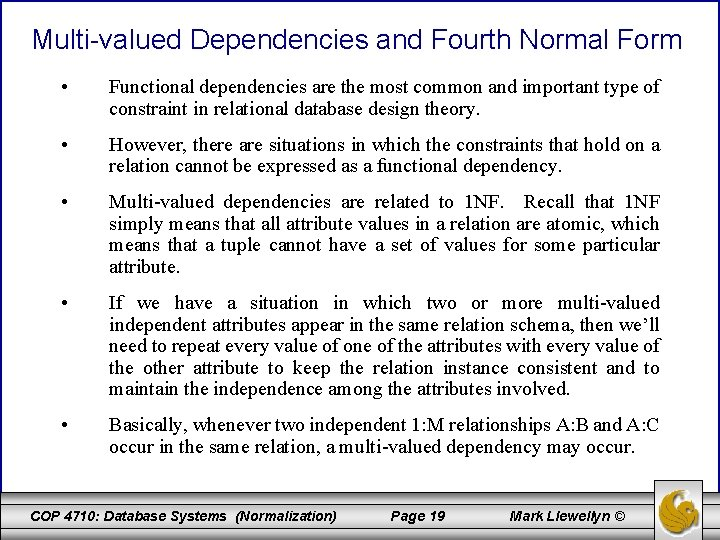Multi-valued Dependencies and Fourth Normal Form • Functional dependencies are the most common and important type of constraint in relational database design theory. • However, there are situations in which the constraints that hold on a relation cannot be expressed as a functional dependency. • Multi-valued dependencies are related to 1 NF. Recall that 1 NF simply means that all attribute values in a relation are atomic, which means that a tuple cannot have a set of values for some particular attribute. • If we have a situation in which two or more multi-valued independent attributes appear in the same relation schema, then we’ll need to repeat every value of one of the attributes with every value of the other attribute to keep the relation instance consistent and to maintain the independence among the attributes involved. • Basically, whenever two independent 1: M relationships A: B and A: C occur in the same relation, a multi-valued dependency may occur. COP 4710: Database Systems (Normalization) Page 19 Mark Llewellyn ©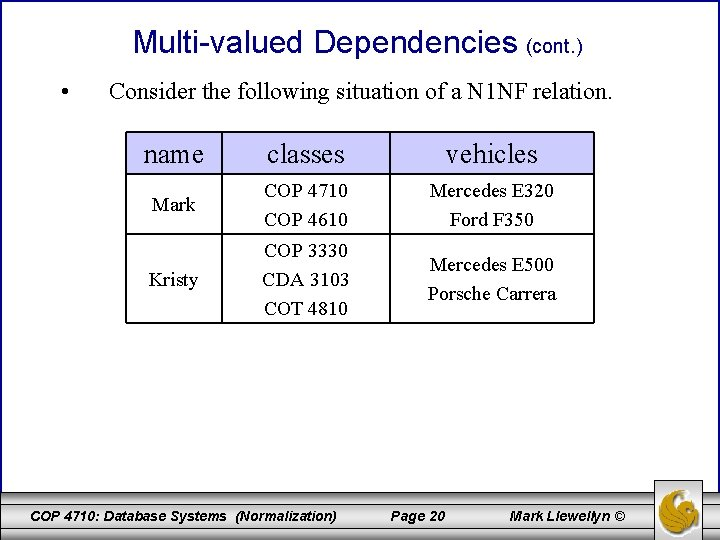Multi-valued Dependencies (cont. ) • Consider the following situation of a N 1 NF relation. name classes vehicles Mark COP 4710 COP 4610 Mercedes E 320 Ford F 350 Kristy COP 3330 CDA 3103 COT 4810 Mercedes E 500 Porsche Carrera COP 4710: Database Systems (Normalization) Page 20 Mark Llewellyn ©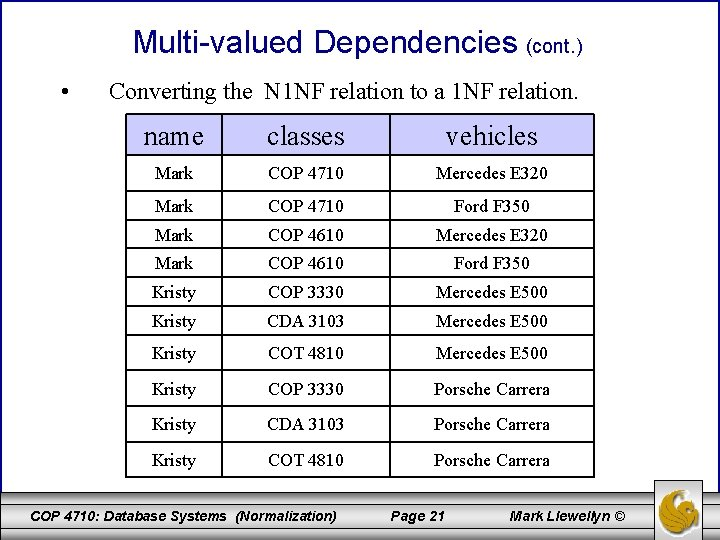Multi-valued Dependencies (cont. ) • Converting the N 1 NF relation to a 1 NF relation. name classes vehicles Mark COP 4710 Mercedes E 320 Mark COP 4710 Ford F 350 Mark COP 4610 Mercedes E 320 Mark COP 4610 Ford F 350 Kristy COP 3330 Mercedes E 500 Kristy CDA 3103 Mercedes E 500 Kristy COT 4810 Mercedes E 500 Kristy COP 3330 Porsche Carrera Kristy CDA 3103 Porsche Carrera Kristy COT 4810 Porsche Carrera COP 4710: Database Systems (Normalization) Page 21 Mark Llewellyn ©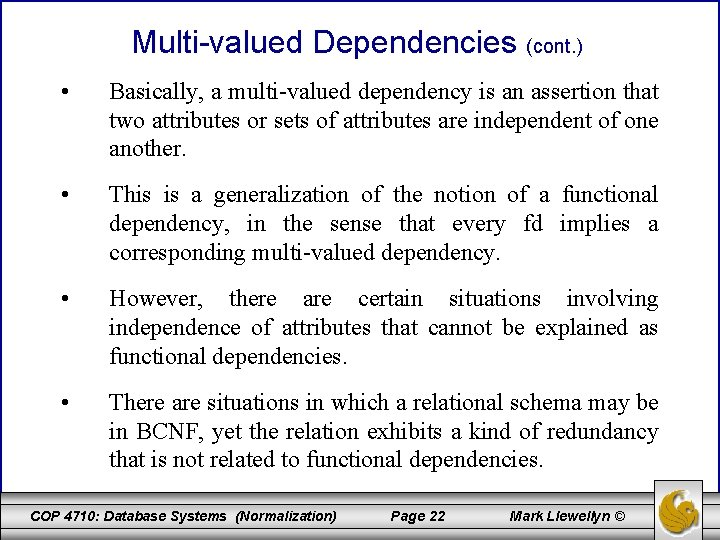Multi-valued Dependencies (cont. ) • Basically, a multi-valued dependency is an assertion that two attributes or sets of attributes are independent of one another. • This is a generalization of the notion of a functional dependency, in the sense that every fd implies a corresponding multi-valued dependency. • However, there are certain situations involving independence of attributes that cannot be explained as functional dependencies. • There are situations in which a relational schema may be in BCNF, yet the relation exhibits a kind of redundancy that is not related to functional dependencies. COP 4710: Database Systems (Normalization) Page 22 Mark Llewellyn ©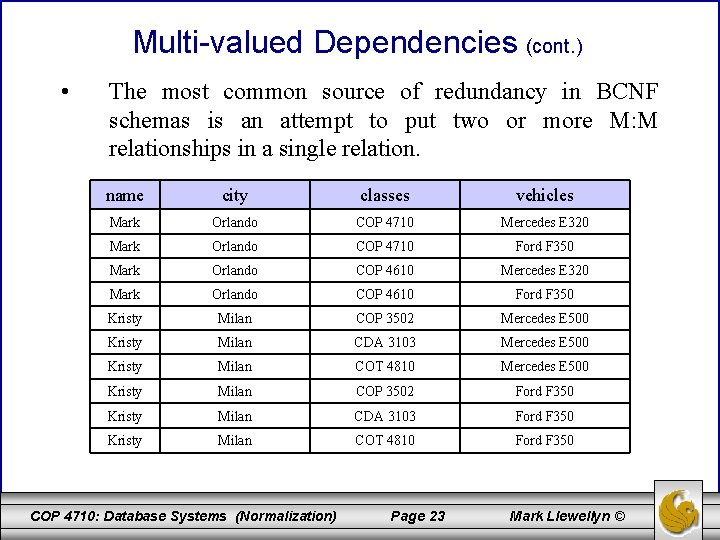Multi-valued Dependencies (cont. ) • The most common source of redundancy in BCNF schemas is an attempt to put two or more M: M relationships in a single relation. name city classes vehicles Mark Orlando COP 4710 Mercedes E 320 Mark Orlando COP 4710 Ford F 350 Mark Orlando COP 4610 Mercedes E 320 Mark Orlando COP 4610 Ford F 350 Kristy Milan COP 3502 Mercedes E 500 Kristy Milan CDA 3103 Mercedes E 500 Kristy Milan COT 4810 Mercedes E 500 Kristy Milan COP 3502 Ford F 350 Kristy Milan CDA 3103 Ford F 350 Kristy Milan COT 4810 Ford F 350 COP 4710: Database Systems (Normalization) Page 23 Mark Llewellyn ©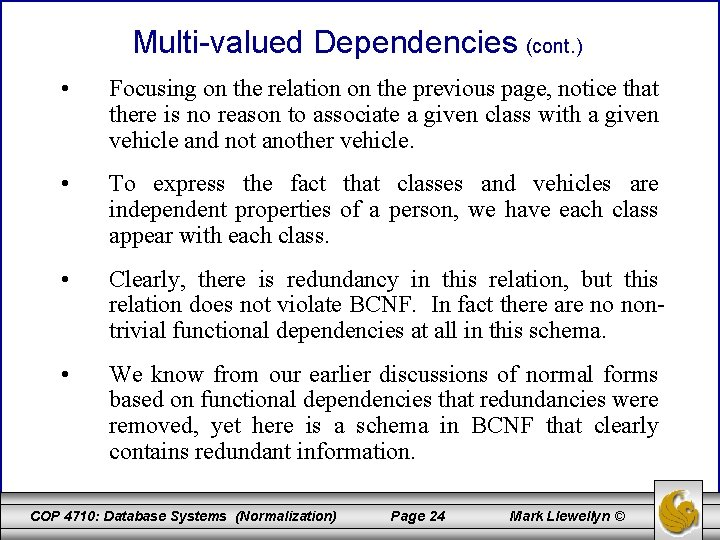Multi-valued Dependencies (cont. ) • Focusing on the relation on the previous page, notice that there is no reason to associate a given class with a given vehicle and not another vehicle. • To express the fact that classes and vehicles are independent properties of a person, we have each class appear with each class. • Clearly, there is redundancy in this relation, but this relation does not violate BCNF. In fact there are no nontrivial functional dependencies at all in this schema. • We know from our earlier discussions of normal forms based on functional dependencies that redundancies were removed, yet here is a schema in BCNF that clearly contains redundant information. COP 4710: Database Systems (Normalization) Page 24 Mark Llewellyn ©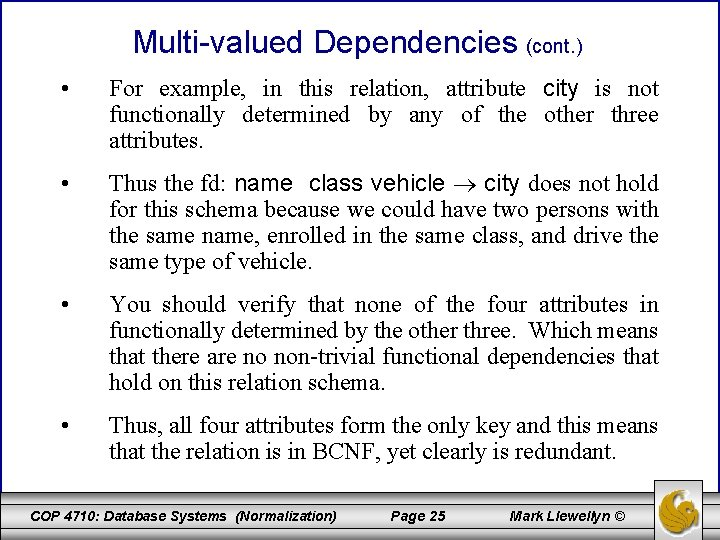Multi-valued Dependencies (cont. ) • For example, in this relation, attribute city is not functionally determined by any of the other three attributes. • Thus the fd: name class vehicle city does not hold for this schema because we could have two persons with the same name, enrolled in the same class, and drive the same type of vehicle. • You should verify that none of the four attributes in functionally determined by the other three. Which means that there are no non-trivial functional dependencies that hold on this relation schema. • Thus, all four attributes form the only key and this means that the relation is in BCNF, yet clearly is redundant. COP 4710: Database Systems (Normalization) Page 25 Mark Llewellyn ©Multi-valued Dependencies (cont. ) • A multi-valued dependency (mvd) is a statement about some relation R that when you fix the values for one set of attributes, then the values in certain other attributes are independent of the values of all the other attributes in the relation. • More precisely, we have the mvd A 1 A 2. . . An ↠ B 1 B 2. . . Bm holds for a relation R if when we restrict ourselves to the tuples of R that have particular values for each of the attributes among the A’s, then the set of values we find among the B’s is independent of the set of values we find among the attributes of R that are not among the A’s or B’s. COP 4710: Database Systems (Normalization) Page 26 Mark Llewellyn ©Multi-valued Dependencies (cont. ) • Even more precisely, a mvd holds if: For each pair of tuples t and u of relation R that agree on all the A’s, we can find in R some tuple v that agrees: 1. With both t and u on the A’s 2. With t on the B’s 3. With u on all attributes of R that are not among the A’s or B’s. – Note that we can use this rule with t and u interchanged, to infer the existence of a fourth tuple w that agrees with u on the B’s and with t on the other attributes. As a consequence, for any fixed values of the A’s, the associated values of the B’s and the other attributes appear in all possible combinations in different tuples. COP 4710: Database Systems (Normalization) Page 27 Mark Llewellyn ©Relationship of Tuple v to Tuple t When mvd Exists A’s B’s others tuple t a 1 b 1 c 1 tuple v a 1 b 1 c 2 tuple u a 1 b 2 c 2 A multi-valued dependency guarantees that tuple v exists COP 4710: Database Systems (Normalization) Page 28 Mark Llewellyn ©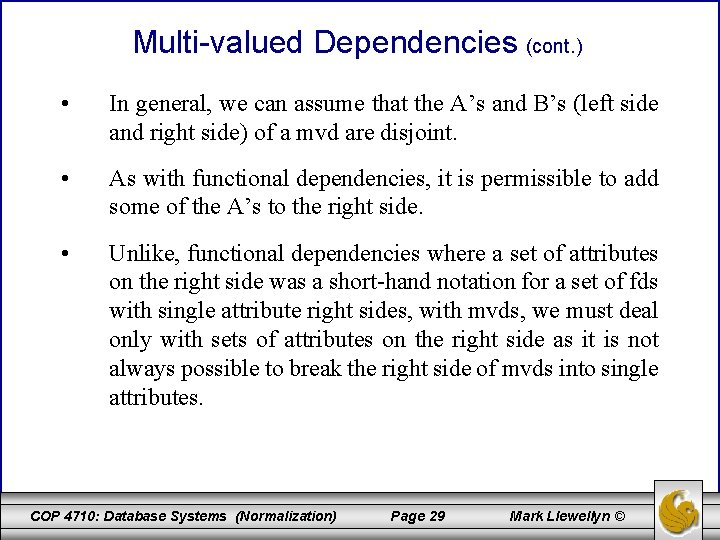Multi-valued Dependencies (cont. ) • In general, we can assume that the A’s and B’s (left side and right side) of a mvd are disjoint. • As with functional dependencies, it is permissible to add some of the A’s to the right side. • Unlike, functional dependencies where a set of attributes on the right side was a short-hand notation for a set of fds with single attribute right sides, with mvds, we must deal only with sets of attributes on the right side as it is not always possible to break the right side of mvds into single attributes. COP 4710: Database Systems (Normalization) Page 29 Mark Llewellyn ©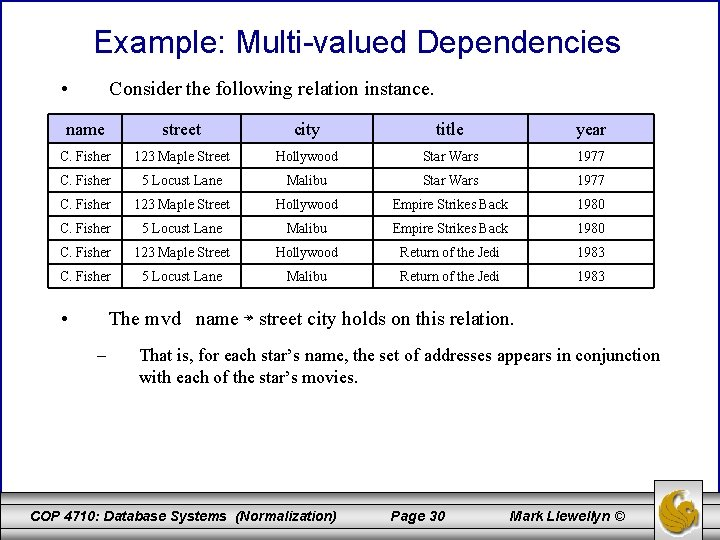Example: Multi-valued Dependencies • Consider the following relation instance. name street city title year C. Fisher 123 Maple Street Hollywood Star Wars 1977 C. Fisher 5 Locust Lane Malibu Star Wars 1977 C. Fisher 123 Maple Street Hollywood Empire Strikes Back 1980 C. Fisher 5 Locust Lane Malibu Empire Strikes Back 1980 C. Fisher 123 Maple Street Hollywood Return of the Jedi 1983 C. Fisher 5 Locust Lane Malibu Return of the Jedi 1983 • The mvd name ↠ street city holds on this relation. – That is, for each star’s name, the set of addresses appears in conjunction with each of the star’s movies. COP 4710: Database Systems (Normalization) Page 30 Mark Llewellyn ©Example: Multi-valued Dependencies (cont. ) • For an example of how the formal definition of this mvd applies, consider the first and fourth tuples from the previous relation instance. name street city title year C. Fisher 123 Maple Street Hollywood Star Wars 1977 C. Fisher 5 Locust Lane Malibu Empire Strikes Back 1980 • If we let the first tuple be t and the second tuple be u, then the mvd asserts that we must also find in R the tuple that has name C. Fisher, a street and city that agree with the first tuple, and other attributes (title and year) that agree with the second tuple. There is indeed such a tuple (the third tuple in the original instance). name street city title year C. Fisher 123 Maple Street Hollywood Empire Strikes Back 1980 COP 4710: Database Systems (Normalization) Page 31 Mark Llewellyn ©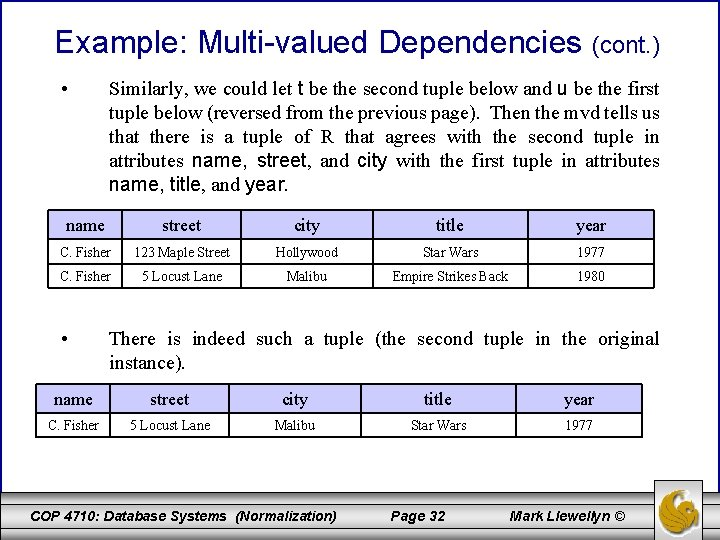Example: Multi-valued Dependencies (cont. ) • Similarly, we could let t be the second tuple below and u be the first tuple below (reversed from the previous page). Then the mvd tells us that there is a tuple of R that agrees with the second tuple in attributes name, street, and city with the first tuple in attributes name, title, and year. name street city title year C. Fisher 123 Maple Street Hollywood Star Wars 1977 C. Fisher 5 Locust Lane Malibu Empire Strikes Back 1980 • There is indeed such a tuple (the second tuple in the original instance). name street city title year C. Fisher 5 Locust Lane Malibu Star Wars 1977 COP 4710: Database Systems (Normalization) Page 32 Mark Llewellyn ©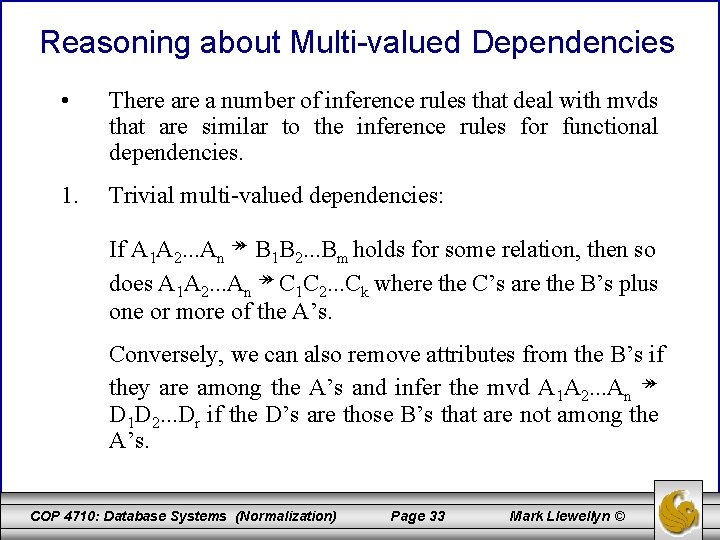Reasoning about Multi-valued Dependencies • There a number of inference rules that deal with mvds that are similar to the inference rules for functional dependencies. 1. Trivial multi-valued dependencies: If A 1 A 2. . . An ↠ B 1 B 2. . . Bm holds for some relation, then so does A 1 A 2. . . An ↠ C 1 C 2. . . Ck where the C’s are the B’s plus one or more of the A’s. Conversely, we can also remove attributes from the B’s if they are among the A’s and infer the mvd A 1 A 2. . . An ↠ D 1 D 2. . . Dr if the D’s are those B’s that are not among the A’s. COP 4710: Database Systems (Normalization) Page 33 Mark Llewellyn ©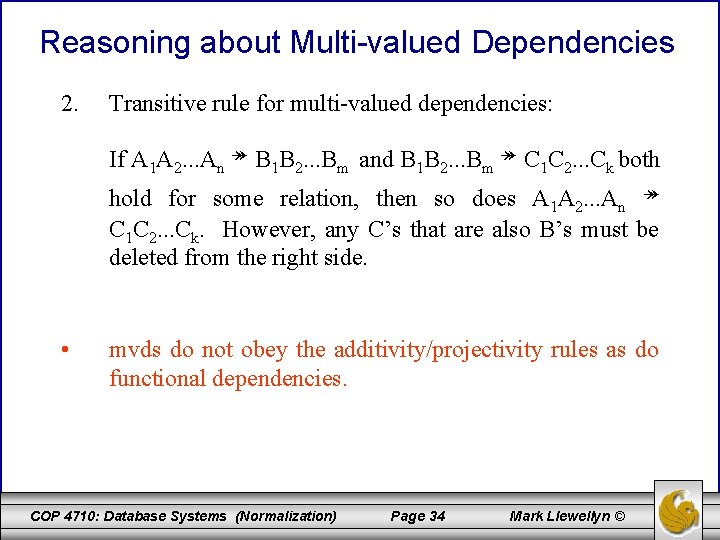Reasoning about Multi-valued Dependencies 2. Transitive rule for multi-valued dependencies: If A 1 A 2. . . An ↠ B 1 B 2. . . Bm and B 1 B 2. . . Bm ↠ C 1 C 2. . . Ck both hold for some relation, then so does A 1 A 2. . . An ↠ C 1 C 2. . . Ck. However, any C’s that are also B’s must be deleted from the right side. • mvds do not obey the additivity/projectivity rules as do functional dependencies. COP 4710: Database Systems (Normalization) Page 34 Mark Llewellyn ©Reasoning about Multi-valued Dependencies • Consider the same relation schema as before, where the mvd name ↠ street city held. If the projectivity (splitting) rule held we would expect that name ↠ street would also be true. This mvd states that each star’s street addresses are independent of the other attributes (including city). However, that statement is false. The first two tuples in the relation instance indicate that this is not true. name street city title year C. Fisher 123 Maple Street Hollywood Star Wars 1977 C. Fisher 5 Locust Lane Malibu Star Wars 1977 COP 4710: Database Systems (Normalization) Page 35 Mark Llewellyn ©Reasoning about Multi-valued Dependencies • This hypothetical mvd name ↠ street, if it held would allow us to infer that the tuples with the streets interchanged would be in the relation instance. However, these tuples are not there because the home at 5 Locust Lane is in Malibu and not Hollywood. name street city title year C. Fisher 5 Locust Lane Hollywood Star Wars 1977 C. Fisher 123 Maple Street Malibu Star Wars 1977 invalid tuples that cannot exist COP 4710: Database Systems (Normalization) Page 36 Mark Llewellyn ©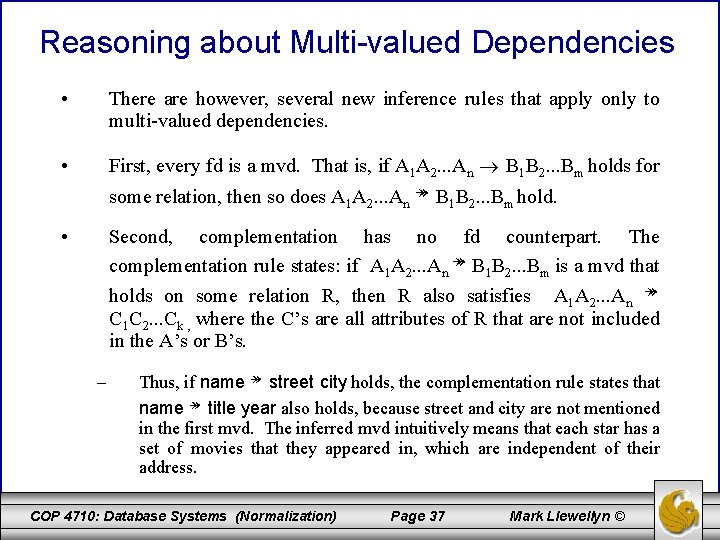Reasoning about Multi-valued Dependencies • There are however, several new inference rules that apply only to multi-valued dependencies. • First, every fd is a mvd. That is, if A 1 A 2. . . An B 1 B 2. . . Bm holds for some relation, then so does A 1 A 2. . . An ↠ B 1 B 2. . . Bm hold. • Second, complementation has no fd counterpart. The complementation rule states: if A 1 A 2. . . An ↠ B 1 B 2. . . Bm is a mvd that holds on some relation R, then R also satisfies A 1 A 2. . . An ↠ C 1 C 2. . . Ck , where the C’s are all attributes of R that are not included in the A’s or B’s. – Thus, if name ↠ street city holds, the complementation rule states that name ↠ title year also holds, because street and city are not mentioned in the first mvd. The inferred mvd intuitively means that each star has a set of movies that they appeared in, which are independent of their address. COP 4710: Database Systems (Normalization) Page 37 Mark Llewellyn ©Fourth Normal Form • The redundancy that we’ve seen in the relation instances in this section of the notes are caused by the existence of multi-valued dependencies. • As we did with functional dependencies, we can use multi-valued dependencies and a different decomposition algorithm to produce a stronger normal form which is based not on functional dependencies but the multivalued dependencies. • Fourth Normal Form (4 NF) eliminates all non-trivial multi-valued dependencies (as are all fds that violate BCNF). The resulting decomposition scheme has neither the redundancy from fds nor redundancy from mvds. COP 4710: Database Systems (Normalization) Page 38 Mark Llewellyn ©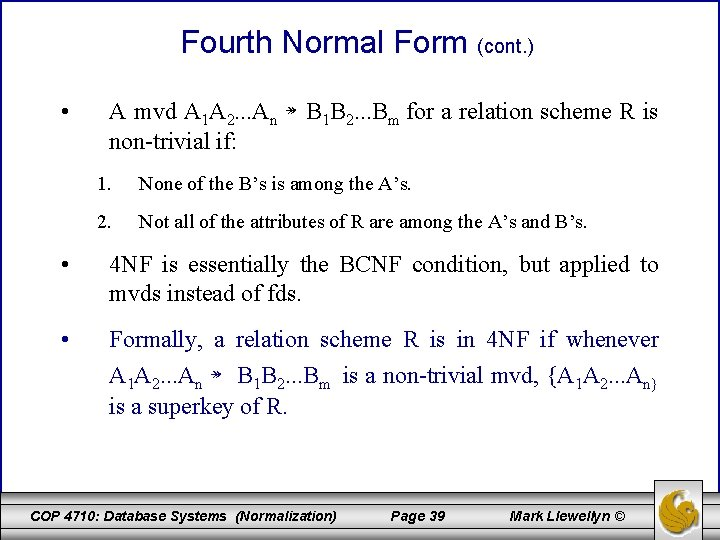Fourth Normal Form (cont. ) • A mvd A 1 A 2. . . An ↠ B 1 B 2. . . Bm for a relation scheme R is non-trivial if: 1. None of the B’s is among the A’s. 2. Not all of the attributes of R are among the A’s and B’s. • 4 NF is essentially the BCNF condition, but applied to mvds instead of fds. • Formally, a relation scheme R is in 4 NF if whenever A 1 A 2. . . An ↠ B 1 B 2. . . Bm is a non-trivial mvd, {A 1 A 2. . . An} is a superkey of R. COP 4710: Database Systems (Normalization) Page 39 Mark Llewellyn ©Fourth Normal Form (cont. ) • The example relation scheme that we have been dealing with is not in 4 NF because name ↠ street city is a nontrivial mvd, yet name by itself is not a superkey. In fact, for this relation the only key is all the attributes. • 4 NF is truly a generalization of BCNF. Since every fd is a mvd, every BCNF violation is also a 4 NF violation. In other words, every relation scheme that is in 4 NF is therefore in BCNF. • However, there are some relation that are in BCNF but not in 4 NF. The relation instance we have been using in this section of notes is a case in point. It is clearly in BCNF, yet as we just illustrated, it is not in 4 NF. COP 4710: Database Systems (Normalization) Page 40 Mark Llewellyn ©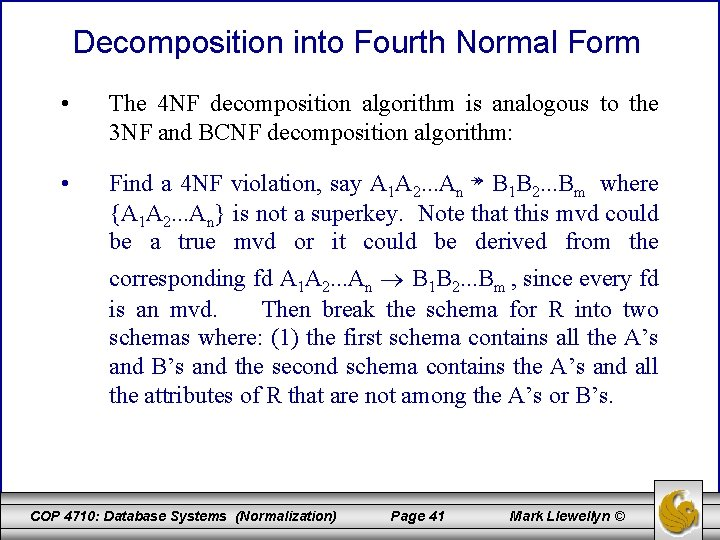Decomposition into Fourth Normal Form • The 4 NF decomposition algorithm is analogous to the 3 NF and BCNF decomposition algorithm: • Find a 4 NF violation, say A 1 A 2. . . An ↠ B 1 B 2. . . Bm where {A 1 A 2. . . An} is not a superkey. Note that this mvd could be a true mvd or it could be derived from the corresponding fd A 1 A 2. . . An B 1 B 2. . . Bm , since every fd is an mvd. Then break the schema for R into two schemas where: (1) the first schema contains all the A’s and B’s and the second schema contains the A’s and all the attributes of R that are not among the A’s or B’s. COP 4710: Database Systems (Normalization) Page 41 Mark Llewellyn ©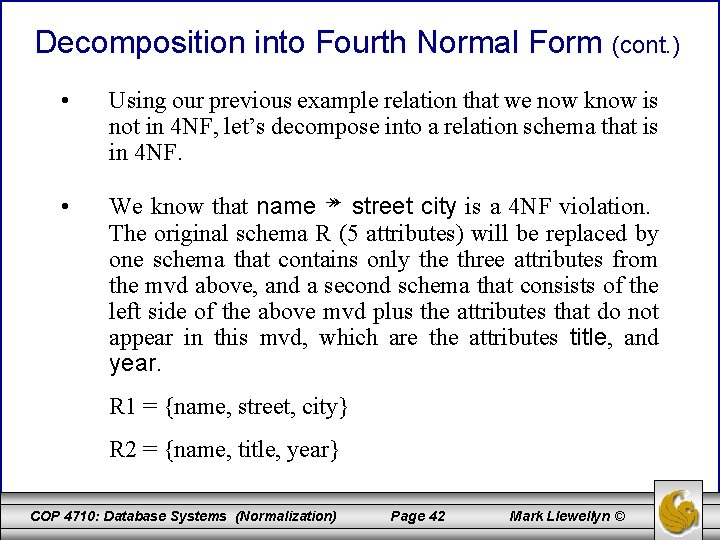Decomposition into Fourth Normal Form (cont. ) • Using our previous example relation that we now know is not in 4 NF, let’s decompose into a relation schema that is in 4 NF. • We know that name ↠ street city is a 4 NF violation. The original schema R (5 attributes) will be replaced by one schema that contains only the three attributes from the mvd above, and a second schema that consists of the left side of the above mvd plus the attributes that do not appear in this mvd, which are the attributes title, and year. R 1 = {name, street, city} R 2 = {name, title, year} COP 4710: Database Systems (Normalization) Page 42 Mark Llewellyn ©Decomposition into Fourth Normal Form (cont. ) R 1 = {name, street, city} • R 2 = {name, title, year} In each of these schema there are no non-trivial mvds or fds, so they are both in 4 NF. Notice that in the relation scheme R 1, the mvd name ↠ street city is now trivial since it involves every attribute. Likewise, in R 2, the mvd name ↠ title year is also trivial. COP 4710: Database Systems (Normalization) Page 43 Mark Llewellyn ©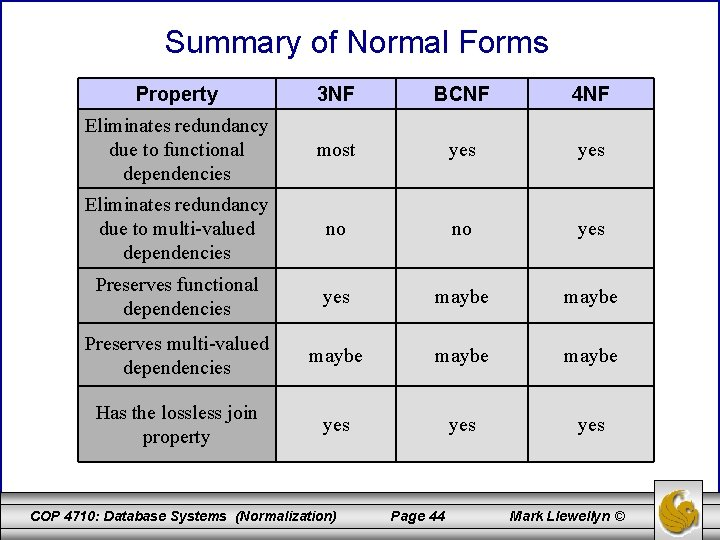Summary of Normal Forms Property 3 NF BCNF 4 NF Eliminates redundancy due to functional dependencies most yes Eliminates redundancy due to multi-valued dependencies no no yes Preserves functional dependencies yes maybe Preserves multi-valued dependencies maybe Has the lossless join property yes yes COP 4710: Database Systems (Normalization) Page 44 Mark Llewellyn ©